# microsoft dp-100 practice test

## Designing and Implementing a Data Science Solution on Azure

##### Question 1 Topic 3, Mixed Questions

DRAG DROP
You need to correct the model fit issue.
Which three actions should you perform in sequence? To answer, move the appropriate actions from the list of actions to the
answer area and arrange them in the correct order.
Select and Place: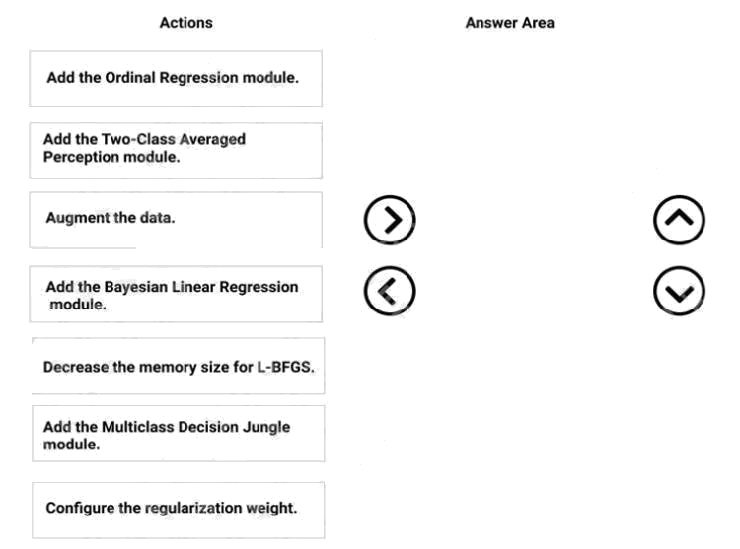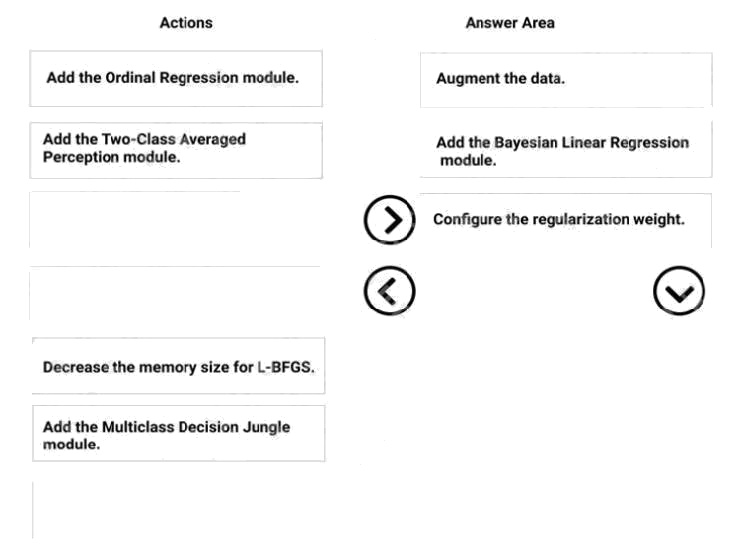Explanation:
Step 1: Augment the data
Scenario: Columns in each dataset contain missing and null values. The datasets also contain many outliers.
Step 2: Add the Bayesian Linear Regression module.
Scenario: You produce a regression model to predict property prices by using the Linear Regression and Bayesian Linear
Regression modules.
Step 3: Configure the regularization weight.
Regularization typically is used to avoid overfitting. For example, in L2 regularization weight, type the value to use as the
weight for L2 regularization. We recommend that you use a non-zero value to avoid overfitting.
Scenario:
Model fit: The model shows signs of overfitting. You need to produce a more refined regression model that reduces the
overfitting.
Multiclass Decision Jungle module:
Decision jungles are a recent extension to decision forests. A decision jungle consists of an ensemble of decision directed
acyclic graphs (DAGs).
L-BFGS:
L-BFGS stands for "limited memory Broyden-Fletcher-Goldfarb-Shanno". It can be found in the wwo-Class Logistic
Regression module, which is used to create a logistic regression model that can be used to predict two (and only two)
outcomes.
References: https://docs.microsoft.com/en-us/azure/machine-learning/studio-module-reference/linear-regr ession

Discussions
0 / 1000

##### Question 2 Topic 3, Mixed Questions

You have a dataset that includes confidential data. You use the dataset to train a model.
You must use a differential privacy parameter to keep the data of individuals safe and private.
You need to reduce the effect of user data on aggregated results.
What should you do?

• A. Decrease the value of the epsilon parameter to reduce the amount of noise added to the data
• B. Increase the value of the epsilon parameter to decrease privacy and increase accuracy
• C. Decrease the value of the epsilon parameter to increase privacy and reduce accuracy
• D. Set the value of the epsilon parameter to 1 to ensure maximum privacy

C

Explanation:
Differential privacy tries to protect against the possibility that a user can produce an indefinite number of reports to eventually
reveal sensitive data. A value known as epsilon measures how noisy, or private, a report is. Epsilon has an inverse
relationship to noise or privacy. The lower the epsilon, the more noisy (and private) the data is.
Reference:
https://docs.microsoft.com/en-us/azure/machine-learning/concept-differential-privacy

Discussions
0 / 1000

##### Question 3 Topic 3, Mixed Questions

You create a binary classification model. The model is registered in an Azure Machine Learning workspace. You use the
Azure Machine Learning Fairness SDK to assess the model fairness.
You develop a training script for the model on a local machine.
You need to load the model fairness metrics into Azure Machine Learning studio.
What should you do?

• B. Implement the create_group_metric_set function
• C. Implement the upload_dashboard_dictionary function
• D. Upload the training script

C

Explanation:
import azureml.contrib.fairness package to perform the upload:
Reference:
https://docs.microsoft.com/en-us/azure/machine-learning/how-to-machine-learning-fairness-aml

Discussions
0 / 1000

##### Question 4 Topic 3, Mixed Questions

HOTSPOT
A biomedical research company plans to enroll people in an experimental medical treatment trial.
You create and train a binary classification model to support selection and admission of patients to the trial. The model
includes the following features: Age, Gender, and Ethnicity.
The model returns different performance metrics for people from different ethnic groups.
You need to use Fairlearn to mitigate and minimize disparities for each category in the Ethnicity feature.
Which technique and constraint should you use? To answer, select the appropriate options in the answer area.
NOTE: Each correct selection is worth one point.
Hot Area: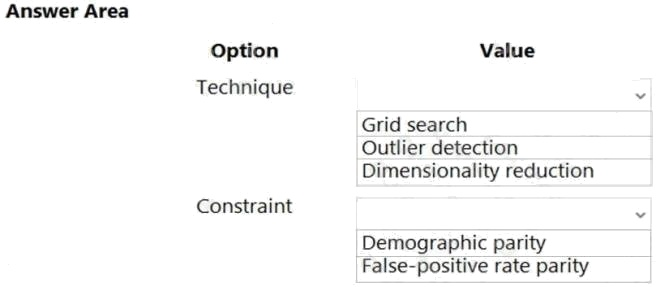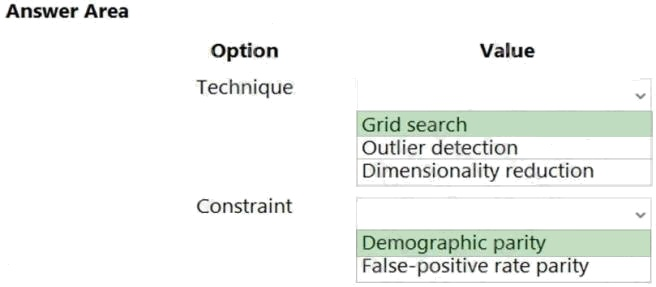Explanation:
Box 1: Grid Search
Fairlearn open-source package provides postprocessing and reduction unfairness mitigation algorithms:
Note: The Fairlearn open-source package provides postprocessing and reduction unfairness mitigation algorithms types:
Reduction: These algorithms take a standard black-box machine learning estimator (e.g., a LightGBM model) and

generate a set of retrained models using a sequence of re-weighted training datasets. Post-processing: These algorithms

take an existing classifier and the sensitive feature as input.
Box 2: Demographic parity
The Fairlearn open-source package supports the following types of parity constraints: Demographic parity, Equalized odds,
Equal opportunity, and Bounded group loss.
Reference:
https://docs.microsoft.com/en-us/azure/machine-learning/concept-fairness-ml

Discussions
0 / 1000

##### Question 5 Topic 3, Mixed Questions

DRAG DROP
You have several machine learning models registered in an Azure Machine Learning workspace.
You must use the Fairlearn dashboard to assess fairness in a selected model.
Which three actions should you perform in sequence? To answer, move the appropriate actions from the list of actions to the
answer area and arrange them in the correct order.
Select and Place: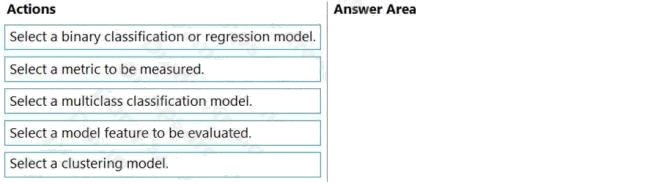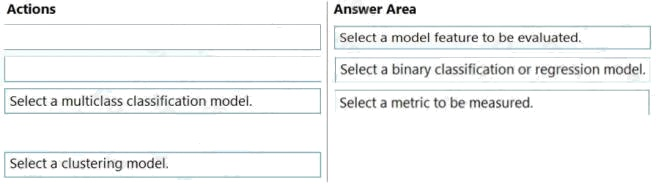Explanation:
Step 1: Select a model feature to be evaluated.
Step 2: Select a binary classification or regression model.
Register your models within Azure Machine Learning. For convenience, store the results in a dictionary, which maps the id of
the registered model (a string in name:version format) to the predictor itself. Example: model_dict = {}
lr_reg_id = register_model("fairness_logistic_regression", lr_predictor) model_dict[lr_reg_id] = lr_predictor
svm_reg_id = register_model("fairness_svm", svm_predictor) model_dict[svm_reg_id] = svm_predictor
Step 3: Select a metric to be measured Precompute fairness metrics.
Create a dashboard dictionary using Fairlearn's metrics package.
Reference:
https://docs.microsoft.com/en-us/azure/machine-learning/how-to-machine-learning-fairness-aml

Discussions
0 / 1000

##### Question 6 Topic 3, Mixed Questions

HOTSPOT
You train a classification model by using a decision tree algorithm.
You create an estimator by running the following Python code. The variable feature_names is a list of all feature names, and
class_names is a list of all class names. from interpret.ext.blackbox import TabularExplainer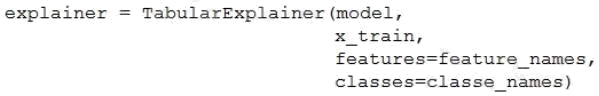You need to explain the predictions made by the model for all classes by determining the importance of all features.
For each of the following statements, select Yes if the statement is true. Otherwise, select No.
NOTE: Each correct selection is worth one point.
Hot Area: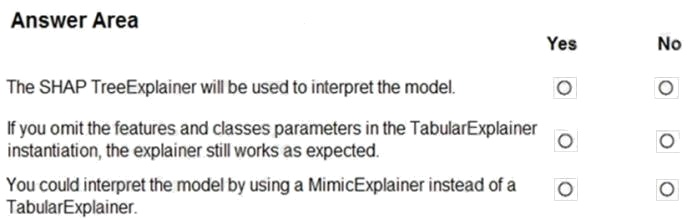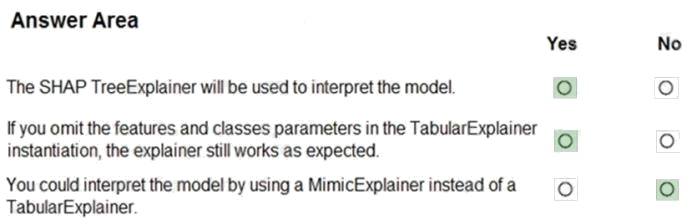Explanation:
Box 1: Yes
TabularExplainer calls one of the three SHAP explainers underneath (TreeExplainer, DeepExplainer, or KernelExplainer).
Box 2: Yes
To make your explanations and visualizations more informative, you can choose to pass in feature names and output class
names if doing classification.
Box 3: No
TabularExplainer automatically selects the most appropriate one for your use case, but you can call each of its three
underlying explainers underneath (TreeExplainer, DeepExplainer, or KernelExplainer) directly.
Reference:
https://docs.microsoft.com/en-us/azure/machine-learning/how-to-machine-learning-interpretability-aml

Discussions
0 / 1000

##### Question 7 Topic 3, Mixed Questions

You are building a binary classification model by using a supplied training set.
The training set is imbalanced between two classes.
You need to resolve the data imbalance.
What are three possible ways to achieve this goal? Each correct answer presents a complete solution.
NOTE: Each correct selection is worth one point.

• A. Penalize the classification
• B. Resample the dataset using undersampling or oversampling
• C. Normalize the training feature set
• D. Generate synthetic samples in the minority class
• E. Use accuracy as the evaluation metric of the model

A B D

Explanation:
A: Try Penalized Models
You can use the same algorithms but give them a different perspective on the problem.
Penalized classification imposes an additional cost on the model for making classification mistakes on the minority class
during training. These penalties can bias the model to pay more attention to the minority class.
B: You can change the dataset that you use to build your predictive model to have more balanced data.
This change is called sampling your dataset and there are two main methods that you can use to even-up the classes:

Consider testing under-sampling when you have an a lot data (tens- or hundreds of thousands of instances or more)

Consider testing over-sampling when you don't have a lot of data (tens of thousands of records or less)
D: Try Generate Synthetic Samples
A simple way to generate synthetic samples is to randomly sample the attributes from instances in the minority class.
Reference:
https://machinelearningmastery.com/tactics-to-combat-imbalanced-classes-in-your-machine-learning-dataset/

Discussions
0 / 1000

##### Question 8 Topic 3, Mixed Questions

Note: This question is part of a series of questions that present the same scenario. Each question in the series contains a
unique solution that might meet the stated goals. Some question sets might have more than one correct solution, while
others might not have a correct solution.
After you answer a question in this section, you will NOT be able to return to it. As a result, these questions will not appear in
the review screen.
You are creating a model to predict the price of a student's artwork depending on the following variables: the student's length
of education, degree type, and art form.
You start by creating a linear regression model.
You need to evaluate the linear regression model.
Solution: Use the following metrics: Mean Absolute Error, Root Mean Absolute Error, Relative Absolute Error, Accuracy,
Precision, Recall, F1 score, and AUC.
Does the solution meet the goal?

• A. Yes
• B. No

B

Explanation:
Accuracy, Precision, Recall, F1 score, and AUC are metrics for evaluating classification models.
Note: Mean Absolute Error, Root Mean Absolute Error, Relative Absolute Error are OK for the linear regression model.
Reference:
https://docs.microsoft.com/en-us/azure/machine-learning/studio-module-reference/evaluate-model

Discussions
0 / 1000

##### Question 9 Topic 3, Mixed Questions

You are a data scientist building a deep convolutional neural network (CNN) for image classification.
The CNN model you build shows signs of overfitting.
You need to reduce overfitting and converge the model to an optimal fit.
Which two actions should you perform? Each correct answer presents a complete solution.
NOTE: Each correct selection is worth one point.

• C. Use training data augmentation.
• D. Reduce the amount of training data.

B D

Explanation:
B: Weight regularization provides an approach to reduce the overfitting of a deep learning neural network model on the
training data and improve the performance of the model on new data, such as the holdout test set.
Keras provides a weight regularization API that allows you to add a penalty for weight size to the loss function.
Three different regularizer instances are provided; they are:
L1: Sum of the absolute weights.

L2: Sum of the squared weights.

L1L2: Sum of the absolute and the squared weights.

D: Because a fully connected layer occupies most of the parameters, it is prone to overfitting. One method to reduce
overfitting is dropout. At each training stage, individual nodes are either "dropped out" of the net with probability 1-p or kept
with probability p, so that a reduced network is left; incoming and outgoing edges to a dropped-out node are also removed.
By avoiding training all nodes on all training data, dropout decreases overfitting.
Reference:
https://machinelearningmastery.com/how-to-reduce-overfitting-in-deep-learning-with-weight-regularization/
https://en.wikipedia.org/wiki/Convolutional_neural_network

Discussions
0 / 1000

##### Question 10 Topic 3, Mixed Questions

HOTSPOT
You are analyzing the asymmetry in a statistical distribution.
The following image contains two density curves that show the probability distribution of two datasets.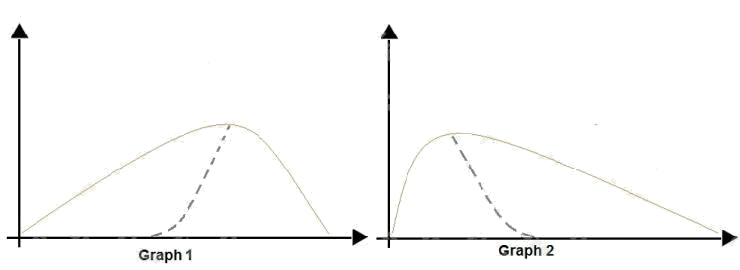Use the drop-down menus to select the answer choice that answers each question based on the information presented in
the graphic.
NOTE: Each correct selection is worth one point.
Hot Area: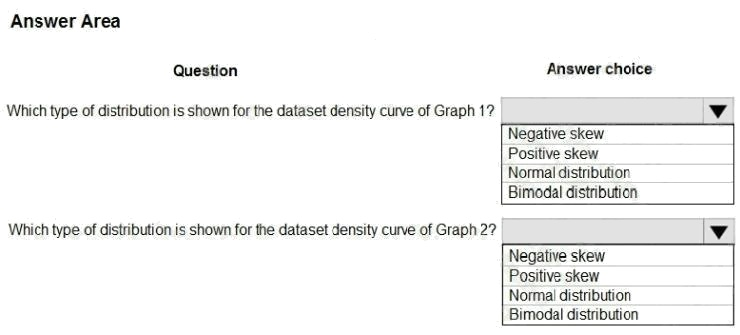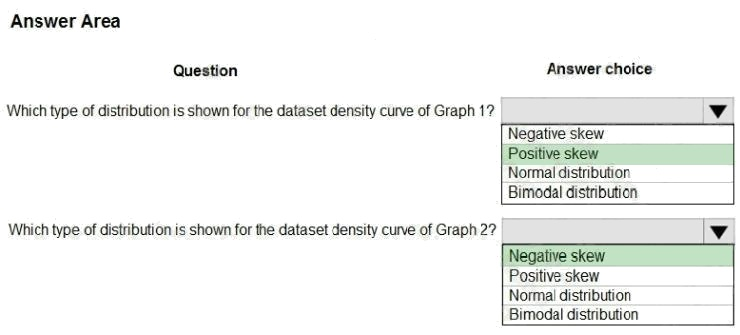Explanation:
Box 1: Positive skew
Positive skew values means the distribution is skewed to the right.
Box 2: Negative skew
Negative skewness values mean the distribution is skewed to the left.
References: https://docs.microsoft.com/en-us/azure/machine-learning/studio-module-reference/compute-elementary-
statistics

Discussions
0 / 1000

##### Question 11 Topic 3, Mixed Questions

DRAG DROP
You have a model with a large difference between the training and validation error values.
You must create a new model and perform cross-validation.
You need to identify a parameter set for the new model using Azure Machine Learning Studio.
Which module you should use for each step? To answer, drag the appropriate modules to the correct steps. Each module
may be used once or more than once, or not at all. You may need to drag the split bar between panes or scroll to view
content.
NOTE: Each correct selection is worth one point.
Select and Place: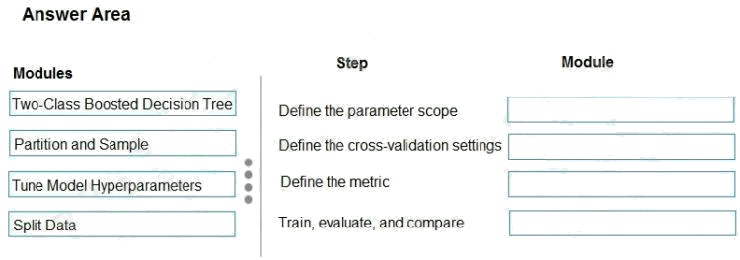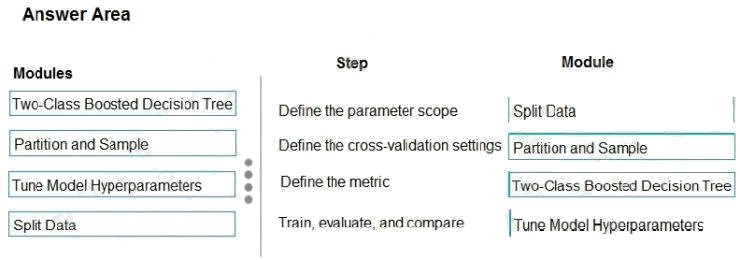Explanation:
Box 1: Split data
Box 2: Partition and Sample
Box 3: Two-Class Boosted Decision Tree
Box 4: Tune Model Hyperparameters
Integrated train and tune: You configure a set of parameters to use, and then let the module iterate over multiple
combinations, measuring accuracy until it finds a "best" model. With most learner modules, you can choose which
parameters should be changed during the training process, and which should remain fixed.
We recommend that you use Cross-Validate Model to establish the goodness of the model given the specified parameters.
Use Tune Model Hyperparameters to identify the optimal parameters.
References: https://docs.microsoft.com/en-us/azure/machine-learning/studio-module-reference/partition-and-sample

Discussions
0 / 1000

##### Question 12 Topic 3, Mixed Questions

HOTSPOT
You are using a decision tree algorithm. You have trained a model that generalizes well at a tree depth equal to 10.
You need to select the bias and variance properties of the model with varying tree depth values.
Which properties should you select for each tree depth? To answer, select the appropriate options in the answer area.
Hot Area: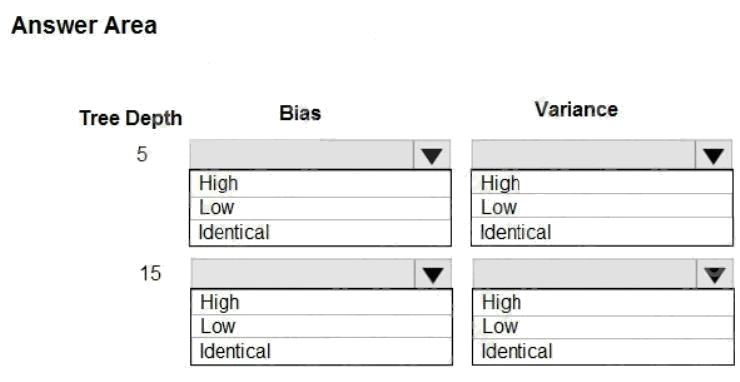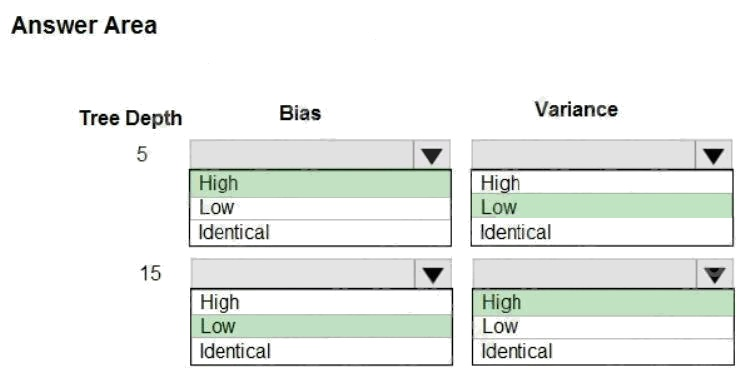Explanation:
In decision trees, the depth of the tree determines the variance. A complicated decision tree (e.g. deep) has low bias and
high variance.
Note: In statistics and machine learning, the biasvariance tradeoff is the property of a set of predictive models whereby
models with a lower bias in parameter estimation have a higher variance of the parameter estimates across samples, and
vice versa. Increasing the bias will decrease the variance. Increasing the variance will decrease the bias.

Discussions
0 / 1000

##### Question 13 Topic 3, Mixed Questions

HOTSPOT
You are developing a linear regression model in Azure Machine Learning Studio. You run an experiment to compare
different algorithms.
The following image displays the results dataset output: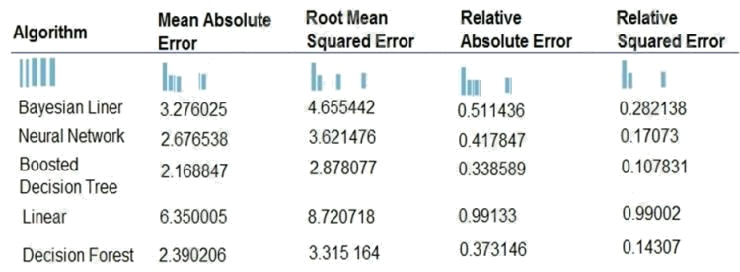Use the drop-down menus to select the answer choice that answers each question based on the information presented in
the image.
NOTE: Each correct selection is worth one point.
Hot Area: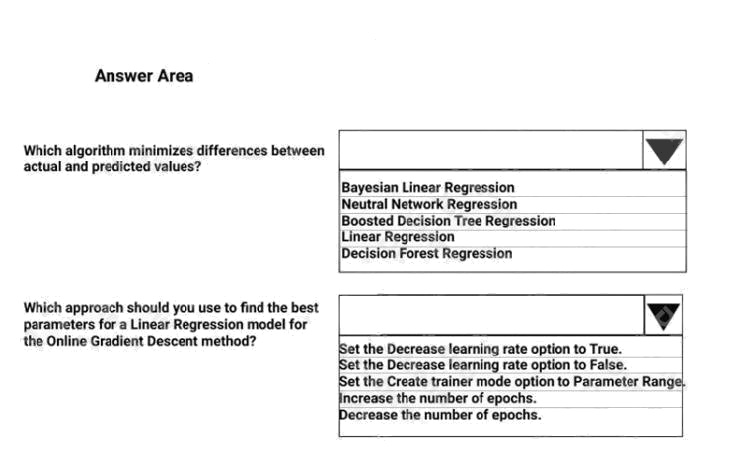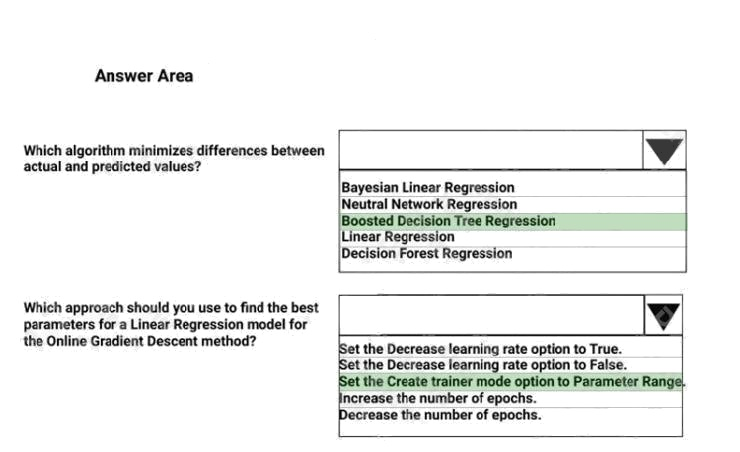Explanation:
Box 1: Boosted Decision Tree Regression
Mean absolute error (MAE) measures how close the predictions are to the actual outcomes; thus, a lower score is better.
Box 2:
Online Gradient Descent: If you want the algorithm to find the best parameters for you, set Create trainer mode option to
Parameter Range. You can then specify multiple values for the algorithm to try.
References: https://docs.microsoft.com/en-us/azure/machine-learning/studio-module-reference/evaluate-model
https://docs.microsoft.com/en-us/azure/machine-learning/studio-module-reference/linear-regression

Discussions
0 / 1000

##### Question 14 Topic 3, Mixed Questions

You are creating a binary classification by using a two-class logistic regression model.
You need to evaluate the model results for imbalance.
Which evaluation metric should you use?

• A. Relative Absolute Error
• B. AUC Curve
• C. Mean Absolute Error
• D. Relative Squared Error
• E. Accuracy
• F. Root Mean Square Error

B

Explanation:
One can inspect the true positive rate vs. the false positive rate in the Receiver Operating Characteristic (ROC) curve and
the corresponding Area Under the Curve (AUC) value. The closer this curve is to the upper left corner; the better the
classifier's performance is (that is maximizing the true positive rate while minimizing the false positive rate). Curves that are
close to the diagonal of the plot, result from classifiers that tend to make predictions that are close to random guessing.
Reference:
https://docs.microsoft.com/en-us/azure/machine-learning/studio/evaluate-model-performance#evaluating-a-binary-
classification-model

Discussions
0 / 1000

##### Question 15 Topic 3, Mixed Questions

You are a data scientist creating a linear regression model.
You need to determine how closely the data fits the regression line.
Which metric should you review?

• A. Root Mean Square Error
• B. Coefficient of determination
• C. Recall
• D. Precision
• E. Mean absolute error

B

Explanation:
Coefficient of determination, often referred to as R2, represents the predictive power of the model as a value between 0 and
1. Zero means the model is random (explains nothing); 1 means there is a perfect fit. However, caution should be used in
interpreting R2 values, as low values can be entirely normal and high values can be suspect.